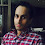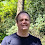## Overview

Using shift in Java and some surprising edge cases.

## Getting the sign

For the following program, what is the values for n so that I can change the type of x without having to change the code.
```// sign is 1 if negative and 0 if non-negative
long sign = x >>> n;
```
There is a value you can replace n such that you don't need to know the type of x

Similarly, I want to shift the lowest bit to be the highest without knowing what type I am shifting

```// sign is MIN_VALUE if odd otherwise 0
long sign = x << n;
```

For bonus points, write formula for all the possible solutions of n (there is more than one)

## Is a power of two

How do you determine an integer is a power of 2.

This is a fairly common interview question. Its pretty hard to figure out in an interview I imagine. To save you googling, you can write this in C with

```x&&!(x&(x-1))
```
In Java, you can write this using just 0 ~ - & == != . Complete this expression using those operators
```long x =
boolean isPow2 = x != 0 .....
```

1.boolean isPow2 = (x != 0) && (x & (x - 1)) == 0;

2.Ok, so now do it without && and where the only number is 0. Hint, you can use ~

3.Getting sign
1. n = 63, works good, cause all type sizes are the power of two. So when >>> operator is used we can simply put the biggest size and in case of smaller types circular shifts are done.
2. n = -1, this works for all types, but there's slight different results for long and other types. For long it would yield Long.MIN_VALUE and for others Integer.MIN_VALUE if odd.

Is a power of two
Just change C expression with provided operators:
boolean isPow2 = (x != 0) & ((x & (x - ((~0)>>>31)))) == 0)

4.A hint: How do you get 1 from 0 using only - and ~

1.Yes, this is much simpler and more clear, thank's.
The updated expression is:
boolean isPow2 = (x != 0) & ((x & (x - (-(~0)))) == 0);

2.See if you can remove some of the ( ) ;)

5.Shifting by 63 and -1 is identical. Shifting by N << 6 + M always shifts by M. For int values its N << 5 + M.